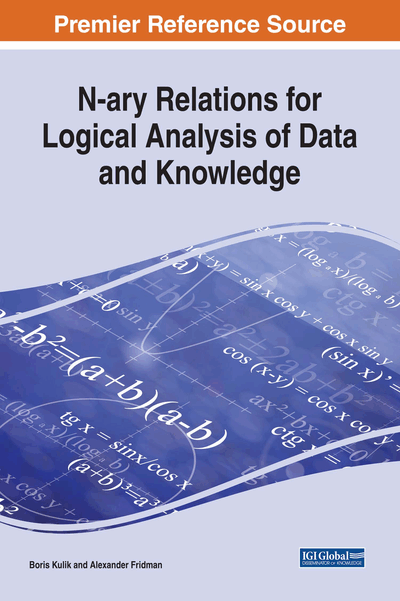# Theoretical Basics of NTA

Copyright: © 2018 |Pages: 28
DOI: 10.4018/978-1-5225-2782-4.ch002
OnDemand PDF Download:
Available
\$37.50
No Current Special Offers

## Abstract

The chapter introduces theoretical features of n-tuple algebra developed by the authors as a theoretical generalization of structures and methods applied in intelligence systems. NTA supports formalization of a wide set of logical problems (abductive and modified conclusions, modeling of graphs, semantic networks, expert rules, etc.). This chapter contains main definitions and theorems of NTA. Unlike relational algebra and theory of binary relations, NTA uses Cartesian product of sets rather than sequences of elements (elementary n-tuples) as a basic structure and implements a general theory of n-ary relations. Novelty of our approach is that we developed some new mathematical structures allowing to implement many techniques of semantic and logical analyses; these methods have no analogies in conventional theories.
Chapter Preview

It is not of the essence of mathematics to be conversant with the ideas of number and quantity. – George Boole

Top

## Introduction

The review presented in Chapter 1 concludes that no general theory of relations has been completed yet. The term “theory of relations” is usually used either for theory of binary relations or for theory of n-ary relations based on relational algebra. In any case, these theories accept the classical mathematical definition of a relation through Cartesian products. If D is a Cartesian product of n different or equal sets, then an n-ary relation R is a certain subset of elementary n-tuples from D. In mathematics, the term “tuple” (or n-tuple) stands for a sequence of some objects. Elementary n-tuple is a sequence of indivisible objects (elements).

Such a definition of n-ary relation allows treating relations as ordinary sets if they are defined on the same Cartesian product D. If so, the complement of an n-ary relation R is the set difference D \ R.

For instance, if D = {1, 2, 3, 4, 5} × {1, 2, 3, 4, 5}, and R is a relation “less than” in the set of numbers {1, 2, 3, 4, 5}, we can get the set of elementary n-tuples corresponding to the relation “more than or equal to” by elimination from D all elementary n-tuples belonging to R.

However, this conformity between algebra of n-ary relations and algebra of sets is no longer valid in totalities of relations defined on various Cartesian products since it is impossible to determine operations of union and intersection for these. Besides, algebra of n-ary relations includes operations of composition and join that have no equivalent operations in algebra of sets.

According to the classical definition, an n-ary relation is a set of elementary n-tuples; however, this definition is not always practical since it results in redundancy due to multiple copying the same data to memory. Let us consider an example relation which reflects the fact that a professor Smith teaches subjects Mathematics, Logic, and Physics: {(Smith, Mathematics), (Smith, Logic), (Smith, Physics)}. This relation can be compacted as a Cartesian product {Smith} × {Mathematics, Logic, Physics}. Obviously, not every relation can be represented as a single Cartesian product composed of non-elementary sets. For instance, the following relation cannot be expressed this way:

.

Nevertheless, every relation can be represented as a union of certain Cartesian products that are, in general case, composed of subsets of corresponding attributes. In the latter example, this union is P = {Smith} × {Mathematics, Logic, Physics} ∪ {Burns} × {Logic, Philosophy}. Transition from elementary n-tuples to tuples composed of sets rather than elements provides a significant reduction in computational resources used for processing relations (calculating unions, intersections, complements, etc.) and data storing.

This chapter introduces n-tuple algebra where Cartesian products of sets are used instead of elementary n-tuples as a basic structure (Kulik, 1995; Kulik, Fridman, & Zuenko, 2012; Kulik, Zuenko, & Fridman, 2010). In our opinion, NTA is a proper means to develop a general theory of n-ary relations. Main relevant NTA-related publications in English are listed in the bottom of the chapter.

## Complete Chapter List

Search this Book:
Reset